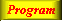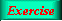Lecture 4 - Page 16 : 34
 Functional Programming in SchemeHigher-order Functions * Introduction to higher-order functions Higher-order functions Some simple and general higher-order functions Linear search in lists Generation of list selectors * Mapping and filtering Classical higher-order functions: Overview Mapping The mapping function Examples of mapping Filtering The filtering function Examples of filtering * Reduction and zipping Reduction The reduction functions Accumulation Zipping The zipping function * Currying The idea of currying Currying in Scheme Examples of currying Ad hoc currying in Scheme (1) Ad hoc currying in Scheme (2) * Web related higher-order functions HTML mirror generation HTML mirror usage examples Making tables with the real mirror Tables with higher-order functions HTML element modifications The function simple-html-table The XHTML mirror in LAML Generation of a leq predicate from enumeration
 The reduction functions
 The function reduce-right is a straightforward recursive function The function reduce-left is a straightforward iterative functionThe function reduce-right.

Notice the fit between the composition of the list and the recursive pattern of the right reduction.The function reduce-left.

There is a misfit between left reduction and the recursive composition of the list with heads and tails. However, an iterative process where we successively combine e1 and e2 (giving r1), r1 and e3 etc., is straightforward. As we have seen several times, this can be done by a tail recursive function, here reduce-help-left.

 Expression Value `(reduce-left - '(1 2 3 4 5))` `-13` `(reduce-right - '(1 2 3 4 5))` `3` `(reduce-left string-append (list "The" " " "End"))` `"The End"` ```(reduce-left append (list (list 1 2 3) (list 'a 'b 'c)))``` `(1 2 3 a b c)`

Examples of reductions. The - left reduction of the list corresponds to calculating the expression (- (- (- (- 1 2) 3) 4) 5). The - right reduction of the list corresponds to calculating the expression (- 1 (- 2 (- 3 (- 4 5)))).Quantifier Functions